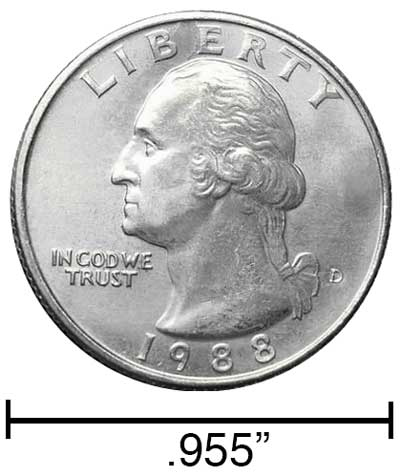# Nanometers to Inches Conversion

Enter the length in nanometers below to get the value converted to inches.

Results in Inches:1 nm = 3.937E-8 in
Hint: use a scientific notation calculator to convert E notation to decimal

## How to Convert Nanometers to InchesTo convert a nanometer measurement to an inch measurement, divide the length by the conversion ratio.

Since one inch is equal to 25,400,000 nanometers, you can use this simple formula to convert:

inches = nanometers ÷ 25,400,000

The length in inches is equal to the nanometers divided by 25,400,000.

For example, here's how to convert 50,000,000 nanometers to inches using the formula above.
50,000,000 nm = (50,000,000 ÷ 25,400,000) = 1.968504"

Our inch fraction calculator can add nanometers and inches together, and it also automatically converts the results to US customary, imperial, and SI metric values.

## Nanometers

One nanometer is equal to one-billionth (1/1,000,000,000) of a meter, which is defined as the distance light travels in a vacuum in a 1/299,792,458 second time interval.

The nanometer, or nanometre, is a multiple of the meter, which is the SI base unit for length. In the metric system, "nano" is the prefix for 10-9. Nanometers can be abbreviated as nm; for example, 1 nanometer can be written as 1 nm.

The nanometer is an extremely small unit of length measurement, often used to measure things that are very small, such as the transistors and electrical pathways in computer processors and nanotechnology.

## Inches

An inch is a unit of linear length measure equal to 1/12 of a foot or 1/36 of a yard. Because the international yard is legally defined to be equal to exactly 0.9144 meters, one inch is equal to 2.54 centimeters.

The inch is a US customary and imperial unit of length. Inches can be abbreviated as in; for example, 1 inch can be written as 1 in.

Inches can also be denoted using the symbol, otherwise known as a double-prime. Often a double-quote (") is used instead of a double-prime for convenience. A double-prime is commonly used to express 1 in as 1″.The standard ruler has 12", and is a common measuring tool for measuring inches. They are also often measured using tape measures, which commonly come in lengths from 6' - 35'. Other types of measuring devices include scales, calipers, measuring wheels, micrometers, yardsticks, and even lasers.

We recommend using a ruler or tape measure for measuring length, which can be found at a local retailer or home center. Rulers are available in imperial, metric, or combination with both values, so make sure you get the correct type for your needs.

Need a ruler? Try our free downloadable and printable rulers, which include both imperial and metric measurements.

## Nanometer to Inch Conversion Table

Nanometer measurements converted to inches
Nanometers Inches
1 nm 0.00000003937"
2 nm 0.00000007874"
3 nm 0.00000011811"
4 nm 0.00000015748"
5 nm 0.00000019685"
6 nm 0.00000023622"
7 nm 0.00000027559"
8 nm 0.00000031496"
9 nm 0.00000035433"
10 nm 0.0000003937"
100 nm 0.000003937"
1,000 nm 0.00003937"
10,000 nm 0.000394"
100,000 nm 0.003937"
1,000,000 nm 0.03937"
10,000,000 nm 0.393701"
100,000,000 nm 3.937"

## References

1. National Institute of Standards and Technology, Checking the Net Contents of Packaged Goods, Handbook 133 - 2019 Edition, https://nvlpubs.nist.gov/nistpubs/hb/2019/NIST.HB.133-2019.pdf